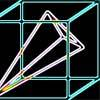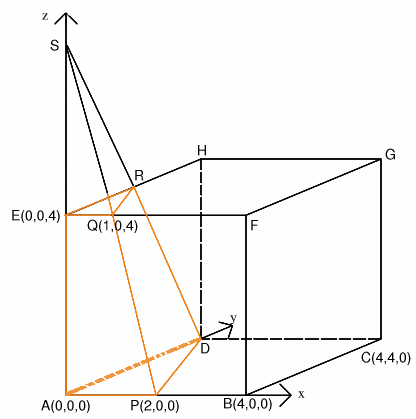#### You may also likeA box of size a cm by b cm by c cm is to be wrapped with a square piece of wrapping paper. Without cutting the paper what is the smallest square this can be?### Four Points on a Cube

What is the surface area of the tetrahedron with one vertex at O the vertex of a unit cube and the other vertices at the centres of the faces of the cube not containing O?### Instant Insanity

We have a set of four very innocent-looking cubes - each face coloured red, blue, green or white - and they have to be arranged in a row so that all of the four colours appear on each of the four long sides of the resulting cuboid.

# Plane to See

##### Age 16 to 18 Challenge Level:

The problem can be solved, without using 3D coordinates, by essentially the same argument as the one used in the solution below. You have to locate the same points, and use similar triangles and the volumes of similar tetrahedra.

This solution came from Brad of Kokomo School, India and a very similar solution fromSue Liu of Madras College, St Andrews.

Using 3D coordinates with point $A$ at the origin, $B$ being the point $(4, 0, 0)$, $C$ the point $(4, 4, 0)$, $D$ the point $(0, 4, 0)$, $E$ the point $(0, 0, 4)$ etc as shown in the diagram. The point $P$ which is the midpoint of $AB$ has coordinates $(2, 0, 0)$ and $Q$, which is a quarter of the way along $EF$ has coordinates $(1, 0, 4)$.The general equation of a plane is $ax + by + cz = 1$ and to find $a$, $b$ and $c$ we only need to know three points in the plane. Hence it is easy to find the equation of the plane through $D$, $P$ and $Q$. Substituting the coordinates of the points into the equation of the plane we get:

$4b = 1$

$2a = 1$

$a + 4c = 1$

This gives $a = 1/2$, $b = 1/4$ and $c = 1/8$. Writing the equation of the plane without fractions we get $$4x + 2y + z = 8.$$ The point $R$ where the plane cuts the line $EH$ has coordinates $(0, y, 4)$ where $0 + 2y + 4 = 8$ so $R$ is the point $(0, 2, 4)$.

Now $RE = 2$, $EQ = 1$, $\angle \rm{ REQ} = 90^\circ$, and DA = 4, AP = 2, $\angle \rm{ DAP} = 90^\circ$ so it follows that the triangles $REQ$ and $DAP$, which lie in parallel planes, are similar. Also, if we extend the lines $AE$, $PQ$ and $DR$ they will meet at one point $S$ where $SE = 4$ and $SA = 8$.

The points $S$, $A$, $P$ and $D$ are the vertices of a tetrahedron which is sliced into two parts by the plane through $R$, $E$ and $Q$. The required volume of the section of the cube is given by subtracting the volume of the tetrahedron $SEQR$ from the volume of the tetrahedron $SAPD$.

Volume of smaller section of cube $= {1\over 3}(32 - 4) = {28\over 3}.$

The volume of the whole cube is $64$ cubic units so the ratio of the volume of the smaller piece to the volume of the cube is $7$ to $48$. The ratio of the volumes of the two pieces of the cube is $7$ to $41$.